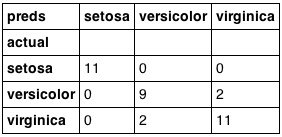# 随机森林（Random Forest）详解（转）

cnblogs.com/maybe2030/p/4585705.html

1 什么是随机森林？

2 随机森林的特点

• 在当前所有算法中，具有极好的准确率/It is unexcelled in accuracy among current algorithms；

• 能够有效地运行在大数据集上/It runs efficiently on large data bases；

• 能够处理具有高维特征的输入样本，而且不需要降维/It can handle thousands of input variables without variable deletion；

• 能够评估各个特征在分类问题上的重要性/It gives estimates of what variables are important in the classification；

• 在生成过程中，能够获取到内部生成误差的一种无偏估计/It generates an internal unbiased estimate of the generalization error as the forest building progresses；

• 对于缺省值问题也能够获得很好得结果/It has an effective method for estimating missing data and maintains accuracy when a large proportion of the data are missing

• ... ...

3 随机森林的相关基础知识

1）信息、熵以及信息增益的概念

I(x)用来表示随机变量的信息，p(xi)指是当xi发生时的概率。

2）决策树

3）集成学习

4 随机森林的生成

1. 如果训练集大小为N，对于每棵树而言，随机且有放回地从训练集中的抽取N个训练样本（这种采样方式称为bootstrap sample方法），作为该树的训练集；

2. 如果每个样本的特征维度为M，指定一个常数m<<M，随机地从M个特征中选取m个特征子集，每次树进行分裂时，从这m个特征中选择最优的；

3. 每棵树都尽最大程度的生长，并且没有剪枝过程。

• 森林中任意两棵树的相关性：相关性越大，错误率越大；

• 森林中每棵树的分类能力：每棵树的分类能力越强，整个森林的错误率越低。

5 袋外错误率（oob error）

1. 对每个样本，计算它作为oob样本的树对它的分类情况（约1/3的树）；

2. 然后以简单多数投票作为该样本的分类结果；

3. 最后用误分个数占样本总数的比率作为随机森林的oob误分率。

oob误分率是随机森林泛化误差的一个无偏估计，它的结果近似于需要大量计算的k折交叉验证。

6 随机森林工作原理解释的一个简单例子

Band 1 : Below $40,000 Band 2:$40,000 – 150,000

Band 3: More than $150,000 随机森林中每一棵树都可以看做是一棵CART（分类回归树），这里假设森林中有5棵CART树，总特征个数N=5，我们取m=1（这里假设每个CART树对应一个不同的特征）。 我们要预测的某个人的信息如下： 1. Age : 35 years ; 2. Gender : Male ; 3. Highest Educational Qualification : Diploma holder; 4. Industry : Manufacturing; 5. Residence : Metro. 根据这五棵CART树的分类结果，我们可以针对这个人的信息建立收入层次的分布情况： 最后，我们得出结论，这个人的收入层次70%是一等，大约24%为二等，6%为三等，所以最终认定该人属于一等收入层次（小于$40,000）。

7 随机森林的Python实现

from sklearn.datasets import load_irisfrom sklearn.ensemble import RandomForestClassifierimport pandas as pdimport numpy as np

df = pd.DataFrame(iris.data, columns=iris.feature_names)
df['is_train'] = np.random.uniform(0, 1, len(df)) <= .75df['species'] = pd.Factor(iris.target, iris.target_names)

train, test = df[df['is_train']==True], df[df['is_train']==False]

features = df.columns[:4]
clf = RandomForestClassifier(n_jobs=2)
y, _ = pd.factorize(train['species'])
clf.fit(train[features], y)

preds = iris.target_names[clf.predict(test[features])]
pd.crosstab(test['species'], preds, rownames=['actual'], colnames=['preds'])import numpy as np
import matplotlib.pyplot as pltfrom matplotlib.colors import ListedColormapfrom sklearn.cross_validation import train_test_splitfrom sklearn.preprocessing import StandardScalerfrom sklearn.datasets import make_moons, make_circles, make_classificationfrom sklearn.neighbors import KNeighborsClassifierfrom sklearn.svm import SVCfrom sklearn.tree import DecisionTreeClassifierfrom sklearn.ensemble import RandomForestClassifier, AdaBoostClassifierfrom sklearn.naive_bayes import GaussianNBfrom sklearn.lda import LDAfrom sklearn.qda import QDA

h = .02  # step size in the mesh

names = ["Nearest Neighbors", "Linear SVM", "RBF SVM", "Decision Tree",         "Random Forest", "AdaBoost", "Naive Bayes", "LDA", "QDA"]
classifiers = [
KNeighborsClassifier(3),
SVC(kernel="linear", C=0.025),
SVC(gamma=2, C=1),
DecisionTreeClassifier(max_depth=5),
RandomForestClassifier(max_depth=5, n_estimators=10, max_features=1),
GaussianNB(),
LDA(),
QDA()]

X, y = make_classification(n_features=2, n_redundant=0, n_informative=2,
random_state=1, n_clusters_per_class=1)
rng = np.random.RandomState(2)
X += 2 * rng.uniform(size=X.shape)
linearly_separable = (X, y)

datasets = [make_moons(noise=0.3, random_state=0),
make_circles(noise=0.2, factor=0.5, random_state=1),
linearly_separable
]

figure = plt.figure(figsize=(27, 9))
i = 1# iterate over datasetsfor ds in datasets:
# preprocess dataset, split into training and test part
X, y = ds
X = StandardScaler().fit_transform(X)
X_train, X_test, y_train, y_test = train_test_split(X, y, test_size=.4)

x_min, x_max = X[:, 0].min() - .5, X[:, 0].max() + .5
y_min, y_max = X[:, 1].min() - .5, X[:, 1].max() + .5
xx, yy = np.meshgrid(np.arange(x_min, x_max, h),
np.arange(y_min, y_max, h))

# just plot the dataset first
cm = plt.cm.RdBu
cm_bright = ListedColormap(['#FF0000', '#0000FF'])
ax = plt.subplot(len(datasets), len(classifiers) + 1, i)
# Plot the training points
ax.scatter(X_train[:, 0], X_train[:, 1], c=y_train, cmap=cm_bright)
# and testing points
ax.scatter(X_test[:, 0], X_test[:, 1], c=y_test, cmap=cm_bright, alpha=0.6)
ax.set_xlim(xx.min(), xx.max())
ax.set_ylim(yy.min(), yy.max())
ax.set_xticks(())
ax.set_yticks(())
i += 1

# iterate over classifiers    for name, clf in zip(names, classifiers):
ax = plt.subplot(len(datasets), len(classifiers) + 1, i)
clf.fit(X_train, y_train)
score = clf.score(X_test, y_test)

# Plot the decision boundary. For that, we will assign a color to each
# point in the mesh [x_min, m_max]x[y_min, y_max].        if hasattr(clf, "decision_function"):
Z = clf.decision_function(np.c_[xx.ravel(), yy.ravel()])        else:
Z = clf.predict_proba(np.c_[xx.ravel(), yy.ravel()])[:, 1]

# Put the result into a color plot
Z = Z.reshape(xx.shape)
ax.contourf(xx, yy, Z, cmap=cm, alpha=.8)

# Plot also the training points
ax.scatter(X_train[:, 0], X_train[:, 1], c=y_train, cmap=cm_bright)
# and testing points
ax.scatter(X_test[:, 0], X_test[:, 1], c=y_test, cmap=cm_bright,
alpha=0.6)

ax.set_xlim(xx.min(), xx.max())
ax.set_ylim(yy.min(), yy.max())
ax.set_xticks(())
ax.set_yticks(())
ax.set_title(name)
ax.text(xx.max() - .3, yy.min() + .3, ('%.2f' % score).lstrip('0'),
size=15, horizontalalignment='right')
plt.show()

1. 从准确率上可以看出，随机森林在这三个测试集上都要优于单棵决策树，90%>85%，82%>80%，95%=95%；

2. 从特征空间上直观地可以看出，随机森林比决策树拥有更强的分割能力（非线性拟合能力）。

posted @ 2017-06-30 10:34  光彩照人  阅读(38541)  评论(2编辑  收藏